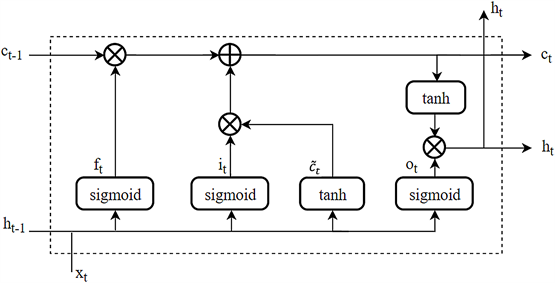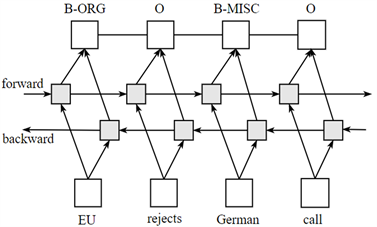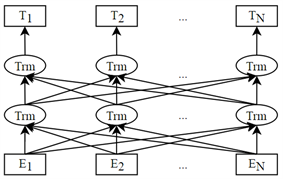﻿ 基于BERT的中文命名实体识别方法

# 基于BERT的中文命名实体识别方法Chinese Named Entity Recognition Method Based on BERT

Abstract: Due to the large differences between Chinese and English, there are a series of challenges in the re-search of Chinese named entity recognition. Currently, the BLSTM-CRF model is the most widely used. The model uses a combination of deep learning models and statistical models for Chinese named entity recognition, which can effectively extract contextual information in the text and con-sider the relationship between tags. However, due to the presence of polysemous characters or words in Chinese, the meaning of the same words in a sentence may be very different. In this case, the performance of the entity recognition of the model is not ideal. In order to better realize that the word representation can not only contain a variety of diversified syntax and semantic representa-tions, but also can model polysemous words, the BERT language model is introduced, which can calculate higher global word vector representations and weights in sentences based on context in-formation. The BERT-BLSTM-CRF named entity recognition model enhances the representation of word vectors through the BERT pre-training model, uses BLSTM to obtain contextual semantic label sequences, and then uses CRF to find the optimal solution. Using the People’s Daily data set to test the proposed model, it can be found that the entity recognition performance of the model is greatly improved compared with the traditional model.

1. 引言

2. 相关工作

3. 命名实体识别模型

3.1. BLSTM模型

LSTM单元结构如图1所示，其中 ${h}_{t-1}$ 为过去隐藏状态， ${h}_{t}$ 为当前隐藏状态， ${c}_{t-1}$ 为过去细胞状态， ${c}_{t}$ 为现在细胞状态， ${x}_{t}$ 为当前输入信息， ${f}_{t}$ 为遗忘门输出， ${i}_{t}$ 为输入门输出， ${\stackrel{˜}{c}}_{t}$ 为候选值向量， ${o}_{t}$ 为输出门的输出。Figure 1. LSTM unit structure

LSTM内存单元具体实现如下：

${f}_{t}=\sigma \left({W}_{f}\left[{h}_{t-1},\text{\hspace{0.17em}}{x}_{t}\right]+{b}_{f}\right)$

${i}_{t}=\sigma \left({W}_{i}\left[{h}_{t-1},\text{\hspace{0.17em}}{x}_{t}\right]+{b}_{i}\right)$

${\stackrel{˜}{c}}_{t}=\mathrm{tanh}\left({W}_{c}\left[{h}_{t-1},\text{\hspace{0.17em}}{x}_{t}\right]+{b}_{c}\right)$

${c}_{t}={f}_{t}\ast {c}_{t-1}+{i}_{t}\ast {\stackrel{˜}{c}}_{t}$

${o}_{t}=\sigma \left({W}_{o}\left[{h}_{t-1},\text{\hspace{0.17em}}{x}_{t}\right]+{b}_{o}\right)$

${h}_{t}={o}_{t}\ast \mathrm{tanh}\left( c t \right)$

LSTM的隐藏状态只包含了过去的信息，但上下文信息对命名实体识别任务来说都十分重要。双向长短时记忆网络(BLSTM)由两个LSTM层组成，基本思想为使用两个LSTM层向前和向后形成独立的隐藏状态，通过正向的LSTM获得上文信息，逆向的LSTM获得下文的信息，然后将两个隐藏状态拼接起来作为最终输出 ，能够高效完成序列标记任务。

3.2. CRF

BLSTM模型只能够预测文本序列与标签的关系，而不能预测标签与标签之间的关系，其原理是输出概率最大值，当输出结果中概率值都很大，就可能会导致输出的两个标签顺序不合理。例如，使用BIO标签策略进行命名实体识别时，正确的标签序列中标签O后面不会出现标签I。

$score\left(L/S\right)=\underset{j=1}{\overset{m}{\sum }}\underset{i=1}{\overset{n}{\sum }}{\lambda }_{j}{f}_{j}\left(S,i,{l}_{i},{l}_{i-1}\right)$

$P\left(L/S\right)=\frac{\mathrm{exp}\left[score\left(L/S\right)\right]}{{\sum }_{{L}^{\prime }}\mathrm{exp}\left[score\left({L}^{\prime }/S\right)\right]}$

3.3. BERT模型Figure 2. BLSTM-CRF model

BLSTM的信息抽取能力弱于Transformer，同时，BLSTM的序列特性使其无法进行并行计算。2018年Devlin等人  提出的BERT (Bidirectional Encoder Representations from Transformers)模型，使用多层Transformer，能够同时获取句子前后两个方向上的信息，在诸多语言模型中取得了更好的成绩。

BERT模型结构如图3所示，它使用了双向Transformer编码器结构，其中编码器框架使用层叠结构，包含多头注意力机制和前馈神经网络 。Figure 3. BERT model structure

Transformer的关键部分是注意力(attention)机制，它通过一个句子中的词与词之间的关联程度调整权重系数矩阵来获取词的表征 ，得到一个有重要性程度区分的输出。Attention机制的计算公式如下：

$Attention\left(Q,K,V\right)=soft\mathrm{max}\left(\frac{Q{K}^{t}}{\sqrt{{d}_{k}}}\right)V$

Transformer模型使用的多头注意力机制可以提高模型在不同位置的注意力单元的不同表示子空间，最终结果是将所有注意力单元的结果整合到一起。公式如下：

$MultiHead\left(Q,K,V\right)=Concat\left(hea{d}_{1},hea{d}_{2},\cdots ,hea{d}_{h}\right){W}^{O}$

$wherehea{d}_{i}=Attention\left(Q{W}_{i}^{Q},K{W}_{i}^{K},V{W}_{i}^{V}\right)$

3.4. BERT-BLSTM-CRF模型Figure 4. BERT-BLSTM-CRF model

4. 实验

4.1. 实验数据

4.2. 评价指标

$P=\frac{识别出的正确实体个数}{识别出的所有实体个数}×100%$

$R=\frac{识别出的正确实体个数}{所有标注的实体个数}×100%$

${F}_{1}=\frac{2PR}{P+R}×100%$

4.3. 模型参数

4.4. 实验结果Table 2. Recognition results of different entities by different modelsTable 3. Named entity recognition results of different models

5. 总结

 Lample, G., Ballesteros, M., Subramanian, S., et al. (2016) Neural Architectures for Named Entity Recognition. Proceedings of the 2016 Conference of the North American Chapter of the Association for Computational Linguistics: Human Language Technologies, San Diego, 12-17 June 2016, 260-270.
https://doi.org/10.18653/v1/N16-1030

 Yang, Z., Salakhutdinov, R. and Cohen, W. (2016) Multi-Task Cross-Lingual Sequence Tagging from Scratch.

 de Oliveira, D.M., Laender, A.H.F., Veloso, A., et al. (2013) FS-NER: A Lightweight Filter-Stream Approach to Named Entity Recognition on Twitter Data. Proceedings of the 22nd International Conference on World Wide Web, Rio de Janeiro, 13-17 May 2013, 597-604.
https://doi.org/10.1145/2487788.2488003

 罗熹, 夏先运, 安莹, 陈先来. 结合多头自注意力机制与BiLSTM-CRF的中文临床实体识别[J]. 湖南大学学报(自然科学版), 2021, 48(4): 45-55.

 王昊, 林克柔, 孟镇, 李心蕾. 文本表示及其特征生成对法律判决书中多类型实体识别的影响分析[J/OL]. 数据分析与知识发现, 1-26.
http://kns.cnki.net/kcms/detail/10.1478.G2.20210326.0959.002.html, 2021-04-25.

 钟诗胜, 陈曦, 赵明航, 张永健. 引入词集级注意力机制的中文命名实体识别方法[J/OL]. 吉林大学学报(工学版), 1-7.
https://doi.org/10.13229/j.cnki.jdxbgxb20200984, 2021-04-26.

 何玉洁, 杜方, 史英杰, 宋丽娟. 基于深度学习的命名实体识别研究综述[J/OL]. 计算机工程与应用, 1-17.
http://kns.cnki.net/kcms/detail/11.2127.TP.20210326.0937.002.html, 2021-04-21.

 王玥. 基于深度学习的命名实体识别研究[D]: [硕士学位论文]. 昆明: 云南财经大学, 2019.

 朱岩, 张利, 王煜. 基于RoBERTa-WWM的中文电子病历命名实体识别[J]. 计算机与现代化, 2021(2): 51-55.

 Collobert, R., Weston, J., Bottou, L., et al. (2011) Natural Language Processing (Almost) from Scratch. Journal of Machine Learning Research, 12, 2493-2537.

 Huang, Z., Xu, W. and Yu, K. (2015) Bidirectional LSTM-CRF Models for Sequence Tagging. arXiv preprint arXiv:1508.01991.

 Chiu, J.P.C. and Nichols, E. (2015) Named Entity Recognition with Bidirectional LSTM-CNNs. Transactions of the Association for Computational Linguistics, 4, 357-370.
https://doi.org/10.1162/tacl_a_00104

 Ma, X. and Hovy, E. (2016) End-to-End Sequence Labeling via Bi-Directional LSTM-CNNs-CRF. Proceedings of the 54th Annual Meeting of the Association for Computational Linguistics (Volume 1: Long Papers), Berlin, 7-12 August 2016, 1064-1074.
https://doi.org/10.18653/v1/P16-1101

 Rei, M., Crichton, G.K.O. and Pyysalo, S. (2016) Attending to Characters in Neural Sequence Labeling Models. Proceedings of COLING 2016, the 26th International Conference on Computational Linguistics: Technical Papers, Osaka, 11-16 December 2016, 309-318.

 王子牛, 姜猛, 高建瓴, 陈娅先. 基于BERT的中文命名实体识别方法[J]. 计算机科学, 2019, 46(S2): 138-142.

 Graves, A. and Schmidhuber, J. (2005) Framewise Phoneme Classification with Bidirectional LSTM and Other Neural Network Architectures. Neural Networks, 18, 602-610.
https://doi.org/10.1016/j.neunet.2005.06.042

 Vaswani, A., Shazeer, N., Parmar, N., et al. (2017) Attention Is All You Need. 31st Conference on Neural Information Processing Systems (NIPS 2017), Long Beach, 4-9 December 2017, 5998-6008.

 Peters, M.E., Neumann, M., Iyyer, M., et al. (2018) Deep Contextualized Word Representations. Proceedings of NAACL-HLT 2018, New Orleans, 1-6 June 2018, 2227-2237.

 Devlin, J., Chang, M.W., Lee, K., et al. (2018) BERT: Pre-Training of Deep Bidirectional Transformers for Language Understanding. Proceedings of NAACL-HLT 2019, Minneapolis, 2-7 June 2019, 4171-4186.

 袁培森, 李润隆, 王翀, 徐焕良. 基于BERT的水稻表型知识图谱中关系抽取研究[J/OL]. 农业机械学报, 1-10.
http://kns.cnki.net/kcms/detail/11.1964.S.20210315.1809.015.html, 2021-04-26.

 谢腾, 杨俊安, 刘辉. 基于BERT-BiLSTM-CRF模型的中文实体识别[J]. 计算机系统应用, 2020, 29(7): 48-55.

Top